Reflecting on Rotations

loopspace

2021-03-26

# Contents

I saw part of a discussion recently which got me re-examining the notions of rotation and reflection. The motivating question could be paraphrased as:

Question 1

Should the operation $x↦-x$ be viewed as a reflection or a rotation?

The answer is, of course, that it depends on what you mean by reflection and rotation. Oh, and by "$x$".

In actual fact, it is the last of these that caught my eye because one of the participants in the discussion wanted the answer to also make sense for functions, which is where it all gets very interesting indeed.

# 1 Defining Terms

The right context for talking about rotations and reflections is geometry. As we're going to include functions, we have to be happy with vector spaces of arbitrary – even infinite – dimension. There's a nice anecdote about how to think of vector spaces of arbitrary dimension: think of ordinary $3$–dimensional space and say "$n$" to yourself in a very firm voice. It's often told with a slightly facetious tone of voice, but it's actually exactly what mathematicians do. So if you're happy with spaces like ${ℝ}^{n}$ or ${ℂ}^{n}$ but less so with the idea of an infinite dimensional space, simply think of ${ℝ}^{n}$ and say "infinity" to yourself in a very firm voice.

Not really much more seriously, a vector is something that behaves like we think a vector ought to behave: namely, you can add, subtract, and scale them1. A vector space is a space of vectors that can all be added to and subtracted from each other – they live together in (relative) harmony. What is slightly weird if you haven't already encountered the notion is that functions $f:ℝ\to ℝ$ are vectors: you can add them, subtract them, and scale them. There are various spaces of such functions, depending on what other conditions we want them to have – such as continuity – and all the usual ones are infinite dimensional.

1It may look like a duck, quack like a duck, but if it doesn't add, subtract, or scale like a duck, it isn't a duck.

To start with, though, we'll deal with finite dimensional spaces. We'll also assume that our spaces come equipped with an inner product, which allows us to talk about lengths and angles in a sensible fashion. So we start with a finite dimensional vector space equipped with the tools for lengths and angles. That's what "$x$" is: a vector in this space.

We'll also need some terminology for the map $x↦-x$. For a given vector space, let $I$ denote the identity transformation on that vector space. Then $x↦-x$ is $-I$.

So what about reflections and rotations? The first thing to note is that both are transformations that preserve lengths and angles2. Such transformations are called orthogonal. But within the space of orthogonal transformations, which are rotations and which reflections?

2One technicality here: we're only considering linear transformations which means that all our reflections and rotations also preserve the origin.

Imagine explaining reflections and rotations to someone who hadn't encountered them before. Rotation, you might say, is what happens when you turn. You start somewhere and turn round: that's a rotation. A reflection, on the other hand, just appears somewhere. There's no way to move yourself from your starting position to your reflection.

And that's the definition we'll use:

Definition 2

A rotation is an orthogonal transformation which can be obtained by a continuous path of orthogonal transformations starting at the identity.

A reflection is an orthogonal transformation which is not a rotation.

I should be clear here: this is the broadest definition of rotation and reflection. There are other definitions which you may encounter which are more restrictive. If you ever encounter someone who insists on using a more restrictive definition, just sprinkle this definition liberally with the word "generalised".

Using this definition, every orthogonal transformation is either a rotation or a reflection. It would be useful to have a way to quickly figure out which. I'm very much of the "down with determinants" school, but I can't deny that they are quite useful in this context.

Lemma 3

In a finite dimensional vector space, an orthogonal transformation $T$ has determinant either $1$ or $-1$ and is a rotation if $\mathrm{det}\left(T\right)=1$, and is a reflection if $\mathrm{det}\left(T\right)=-1$.

Question 1 can then be answered because in a finite dimensional vector space of dimension $n$, $\mathrm{det}\left(-I\right)=\left(-1{\right)}^{n}$. Therefore it is a reflection if $n$ is odd and a rotation if $n$ is even.

# 2 Promotion to a Higher Plane

The original context was $-I:x↦-x$ on numbers, which forms a $1$–dimensional vector space. Therefore the answer to the original question is "reflection". But there is an argument to be made for "rotation", wherein the real line is rotated out of itself and back onto itself, as in Figure 1.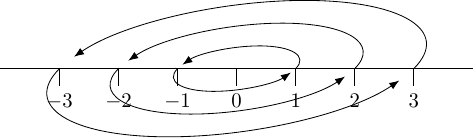Figure 1: Rotating the Real Line

The key here is that we need an extra direction to rotate into. This can be generalised: if $V$ is a vector space then we can add a direction and "promote" reflections in $V$ to rotations in this bigger space. The notation for this bigger space is $V\oplus ℝ$ (think: $V$ plus one more direction). Formally, given an orthogonal transformation $T$ on $V$, we define ${T}^{+}$ on $V\oplus ℝ$ by:

 ${T}^{+}\left(v,\lambda \right)=\left(Tv,\mathrm{det}\left(T\right)\lambda \right)$

Then $\mathrm{det}\left({T}^{+}\right)=\mathrm{det}\left(T\right)×\mathrm{det}\left(T\right)=\mathrm{det}\left(T{\right)}^{2}=1$. So ${T}^{+}$ is always a rotation.

Thus providing we are happy to make our space a bit bigger, we can always think of $-I$ as a rotation3.

3This "adding a dimension" technique is actually something quite important, particularly in Algebraic Topology. Sometimes we want to study what stays the same and what changes when we keep doing it. Stuff that "stays the same" is called stable, so we might be tempted to say that stably all orthogonal transformations are rotations, except that when done properly then the stabilisation of a transformation is obtained via $\left(v,\lambda \right)↦\left(T\left(v\right),\lambda \right)$ which keeps reflections as reflections.

The story doesn't finish here, indeed we're only just getting started. We've seen that when we look at the original context then $-I$ is a rotation or reflection depending on the dimension of the space, but that if we're willing to expand its context we can always force it to be a rotation.

The next stage is to focus on the distinction between merely knowing that $-I$ is a rotation and actually seeing it. That is to say, if $-I$ is a rotation then there is a path through orthogonal transformations from $I$ to $-I$, but how easy is it to find such a path? In the next sections we will examine this as that will help considerably when we turn to functions.

# 3 Finding the Path

There's a moment in every "coming of age" story where the protagonist is told to "visualise their goal and the path will become clear".

Imagine standing at the north pole and wanting to travel to the south pole. Even if we insist on travelling in a straight line at a constant speed, any direction will do. So there isn't a preferred choice of path from the north to the south.

It's the same with travelling from the identity transformation $I$ to $-I$ (assuming we're in a space where that transformation is a rotation). The standard method of finding a path works as follows: pair up the coordinates and in each pair, apply a standard half turn rotation. So in a $4$–dimensional space, the path matrix would look like:

 $\left[\begin{array}{cccc}\mathrm{cos}\left(\theta \right)& -\mathrm{sin}\left(\theta \right)& 0& 0\\ \mathrm{sin}\left(\theta \right)& \mathrm{cos}\left(\theta \right)& 0& 0\\ 0& 0& \mathrm{cos}\left(\theta \right)& -\mathrm{sin}\left(\theta \right)\\ 0& 0& \mathrm{sin}\left(\theta \right)& \mathrm{cos}\left(\theta \right)\end{array}\right]$

The problem is that there are many ways of pairing up the coordinates, and indeed many ways of choosing the coordinates in the first place.

So whether we are in an even dimensional space to begin with, or promote our odd dimensional space to one, actually exhibiting $-I$ as a rotation by finding a path is not a natural thing.

# 4 Doubling Up

There's another way to make odd numbers even, and it has the advantage that it also can be applied to even numbers and they stay even: doubling. Rather than adding a single extra dimension, we simply double all of what's there.

This still fits with the image of rotating the real line because $1+1$ is the same as $2×1$, so doubling $1$ has the same effect as adding one to it, meaning that when generalising Figure 1 we should consider both strategies to see which is the "right" generalisation.

The advantage of doubling is that when pairing up directions, each of the original directions has a natural pair: its copy in the double. So if we denote a vector in the original by $v$ and its copy by ${v}_{i}$, the rotation from $v$ to $-v$ is given by:

 $v↦\mathrm{cos}\left(\theta \right)v+\mathrm{sin}\left(\theta \right){v}_{i}$

The notation is deliberate: this is secretly complex numbers. Instead of regarding our vector space as a real vector space, we augment it to a complex one. This means that as well as scaling by a real scale factor, we can scale by a complex one. And since in the complex plane we can travel from $1$ to $-1$ without passing $0$, we can do so in any complex vector space.

So the notion of $-I$ as a rotation yields a natural path towards complex numbers.

(In fact, in a complex vector space, there are no "reflections" by our criteria: every unitary4 transformation can be connected by a continuous path to the identity.)

4The generalisation of "orthogonal"

# 5 Complex Structure

Once we have introduced the concept of complex numbers, we can see that our first approach to thinking of $-I$ as a rotation is also secretly introducing complex numbers into the mix.

Let's start with the simplest case: ${ℝ}^{2}$, otherwise known as the Euclidean plane. One way to view the process of picking a path of rotations from the identity round to $-I$ is to imagine starting at $\left(\begin{array}{c}1\\ 0\end{array}\right)$ and looking up the directions to $\left(\begin{array}{c}-1\\ 0\end{array}\right)$ in your favourite mapping app. The quickest route would be to head down the $x$–axis, but that's not allowed because we have to stay the same distance from the origin throughout. So we have to travel around the circle. This gives us essentially two routes: clockwise and anticlockwise. Once we make that choice, we apply it to all starting points so that the path from the identity to $-I$ sweeps all points round in the same direction.

Let's consider the transformation which maps a vector $x$ to the half-way point, and call this $J$. That's half-way between $I$ and $-I$. In particular, starting at $\left(\begin{array}{c}1\\ 0\end{array}\right)$ and travelling half-way round leads us to either $\left(\begin{array}{c}0\\ 1\end{array}\right)$ or $\left(\begin{array}{c}0\\ -1\end{array}\right)$ depending on the direction. The crucial property of this $J$ is that if we apply it twice we do the full rotation from $I$ to $-I$ and so ${J}^{2}=-I$.

The next step is to think of $J$ as "multiplication by $i$". It allows us to make our Euclidean plane into the Argand plane by telling us how to multiply by $i$.

This extends: in an even dimensional vector space, it is possible to find an operator $J$ such that ${J}^{2}=-I$ and then this defines a path from $I$ to $-I$ via:

 $\mathrm{cos}\left(\theta \right)v+\mathrm{sin}\left(\theta \right)J\left(v\right)$

The operator $J$, and operators like it, are interesting enough to get their own name.

Definition 4

A linear transformation $J$ on a real vector space with the property that ${J}^{2}=-I$ is called a complex structure.

So if we can find a complex structure, we can find a path from $I$ to $-I$ and thus exhibit $-I$ as a rotation.

# 6 The Story of Functions

What really interested me in this was the almost postscript "What about functions?" tacked on to the end of the original discussion. A lot of the above story makes sense for functions, in particular the part about viewing real stuff as secretly embedded in complex stuff5. One commentator on the original discussion said that they viewed the rotation of $f\left(x\right)$ to $-f\left(x\right)$ as being like rotating the graph about the $x$–axis. In this setting, we no longer view $f$ as a function into $ℝ$ but into $ℂ$ and rotate it within $ℂ$, as in Figure 2.

5Complex stuff is often actually simpler than real stuff; I used to give a talk where I'd say "At this point, I want to complexify everything in sight to simplify it".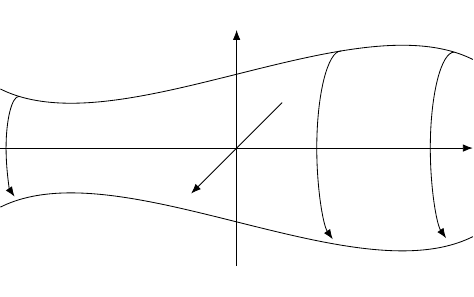Figure 2: Rotating a function

But the first part of the story is more interesting when it comes to function spaces. In that, we figured that $-I$ would be a rotation in an even dimensional space and to exhibit it as such involves finding a complex structure.

There's good news and bad news. The good news is that infinity is even. The bad news is that there is a lot of our discussion which doesn't generalise well to infinite dimensions. As a simple, but ultimately not crucial, example, the notion of a "determinant" only makes sense for very special transformations on infinite dimensional spaces. And the transformation $-I$ is not special in that sense.

More importantly, any finite dimensional real vector space can be made to look like ${ℝ}^{n}$ without too much difficulty and once we know that it looks like ${ℝ}^{n}$ we can easily pair up directions to define a complex structure (if $n$ is even). In infinite dimensions, spaces like ${ℝ}^{n}$ are quite rare. And with function spaces, it can be easier to look for an operator $J$ directly rather than start with directions.

Having said that, some situations are easy. If we take all functions then we don't have any special properties so we can simply pick directions with wild abandon. At the other end of the spectrum, if we take square-integrable functions then this forms what is called a Hilbert space, and these are among the nicest possible spaces in existence6. Picking directions here is child's play, and once we have the directions chosen we can pair them up with impunity and know that everything will Just Work.

6Only superseded by nuclear spaces which have a much cooler name.

So the interesting cases lie somewhere in the middle of these. I think that a particularly intriguing one is that of continuous functions, and so that's what I'm going to look at now. I ought to be flinging words like "topology" around here (beyond that the functions are themselves continuous), but I won't: if you know, be assured that this works.

The space of continuous functions on $ℝ$ is written $C\left(ℝ,ℝ\right)$. What we want to do is to make this into a complex vector space without adding anything to it (so in particular, not converting it to $C\left(ℝ,ℂ\right)$). We'll do that by finding a complex structure $J$ with ${J}^{2}=-I$.

To motivate the next part, let us note that in $ℂ$, we also have choices of "real" and "imaginary" directions and the multiplication by $i$ takes the real to the imaginary and vice versa. So if we can pick out "real" and "imaginary" directions in $C\left(ℝ,ℝ\right)$ we might be on to something. The "real" and "imaginary" must each be "half" of $C\left(ℝ,ℝ\right)$ in that it should be possible to think of every function as being the unique sum of its "real" and "imaginary" parts7.

7Finding the real and imaginary directions is actually stronger than finding a complex structure, so if we can't do it then all might not be lost.

There's an almost obvious split that almost works: take functions that are non-zero only on positive numbers to be "real" and non-zero only on negative numbers to be "imaginary". Then we split a function into its "real" and "imaginary" parts by looking at its restriction to positive and negative numbers respectively.

The reason that this only almost works is because of that pesky point $0$. If we have two functions, one which is zero for negative numbers and one that is zero for positive numbers, then by continuity, each is zero at $0$ and so their sum has to be zero at $0$.

This means that this would work for the subspace of functions which are $0$ at $0$. Given such a function $f$, we can define its real and imaginary parts to be:

 $\begin{array}{rl}{f}_{r}\left(x\right)& =\left\{\begin{array}{ll}f\left(x\right)& x\ge 0\\ 0& x<0\end{array}\\ {f}_{i}\left(x\right)& =\left\{\begin{array}{ll}0& x>0\\ f\left(x\right)& x\le 0\end{array}\end{array}$

Then $f={f}_{r}+{f}_{i}$. The operator $J$ is defined in such a way that it takes a "real" function to the obvious "imaginary" one, and an "imaginary" one to the negative of its corresponding "real" one. If we define $sign\left(x\right)$ to be the sign of $x$:

 $sign\left(x\right)=\left\{\begin{array}{ll}1& x>0\\ 0& x=0\\ -1& x<1\end{array}$

then $J$ is defined by:

 $J\left(f\right)\left(x\right)=\left(-1{\right)}^{sign\left(-x\right)}f\left(-x\right)$

As mentioned above, this only works for the space of functions that are zero at zero. This leaves us with a single left-over direction, which looks as though it puts us in the position of an odd-dimensional vector space from earlier. But fortunately, we can strip off dimensions from our "real" and "imaginary" to match up against this left-over direction.

There's an old Maths joke8 which explains why horses have an infinite number of legs: a horse, so the joke goes, has two hindlegs and forelegs. That makes six legs in total, which is an even number but is also a very odd number of legs for a horse. The only number which is odd and even is infinity, and therefore a horse has an infinite number of legs.

8And therefore not a very good one …

The relevance for us is that infinity is both odd and even, by which I mean that it can be divided into two (so is even) but also if I remove an element then it can still be divided into two (so is odd).

So we have to find a direction in one of our real or imaginary parts of $C\left(ℝ,ℝ\right)$ to match with $0$, let's say $1$. But as we've removed it from the real part, we also need to remove its counterpart from the imaginary part so that the real and imaginary subspaces of $C\left(ℝ,ℝ\right)$ match up, so we also need to separate out $-1$. But then we need to remove a direction to match with $-1$, let's say $-2$, and its counterpart, namely $2$.

Continuing, we need to remove all of $ℤ$. By removing each point, we've remained balanced so our real and imaginary parts still match up. But by removing an infinite number of points we can now pair them up in a different fashion to provide a complex structure.

The last bit is to take an arbitrary continuous function and force it to be zero at the integers. To do this, we need a family of "bump" functions. That is, we need continuous functions9 ${\alpha }_{k}$ with ${\alpha }_{k}\left(k\right)=1$ and ${\alpha }_{k}\left(x\right)=0$ away from $k$. A specific example for ${\alpha }_{0}$ is given in Figure 3 and we can take translates of this for the ${\alpha }_{k}$.

9In all that follows, to adapt the story to other classes of functions, such as smooth, all we need to do is pick suitable bump functions.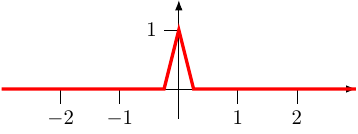Figure 3: A Bump Function

So for a continuous function $f:ℝ\to ℝ$, we start by subtracting suitable multiples of the ${\alpha }_{k}$ to force it to be zero on $ℤ$:

 $f↦{f}_{ℤ}:-f-{\sum }_{k\in ℤ}f\left(k\right){\alpha }_{k}$

This function can then be split into "real" and "imaginary" parts since it is, in particular, zero at zero.

The last step is to declare half of the ${\alpha }_{k}$ to be "real" and half to be "imaginary". The simplest way is via the odd/even split. The full split for the original function $f$ is then:

 $f=\left({f}_{ℤ,r}+{\sum }_{k\in ℤ}f\left(2k\right){\alpha }_{2k}\right)+\left({f}_{ℤ,i}+{\sum }_{k\in ℤ}f\left(2k+1\right){\alpha }_{2k+1}\right)$

This is a little bit messy. We can clean it up a little bit by noting that when we force a function to be zero at all integers, we are effectively splitting it into an infinite number of nice segments that can be separated and recombined without worrying about the continuity of the resulting function. Each segment relates to our original function on a particular interval of the form $\left[k,k+1\right]$, so we have one for each integer. In our complex structure, we link the segment on $\left[k,k+1\right]$ with the segment on its negative counterpart, $\left[-k-1,-k\right]$. But when we look at the values of the function on $ℤ$, we link $k$ with either $k+1$ or $k-1$ (depending on parity). So we have two different schemes in play for pairing up integers. Maybe if we settle for just one we might get a cleaner structure.

So for $f\in C\left(ℝ,ℝ\right)$ and $k\in ℤ$, define ${f}_{k}\in C\left(ℝ,ℝ\right)$ by:

 ${f}_{k}\left(x\right)=\left\{\begin{array}{ll}f\left(k\right)\left(4x-4k+1\right)& k-\frac{1}{4}\le x\le k\\ f\left(x\right)& k\le x\le k+\frac{3}{4}\\ f\left(x\right)-f\left(k+1\right)\left(4x-4k-3\right)& k+\frac{3}{4}\le x\le k+1\\ 0& \text{otherwise}\end{array}$

See Figure 4 for an example of what this looks like. This is determined by the values of $f$ only in the interval $\left[k,k+1\right]$ but maintains continuity.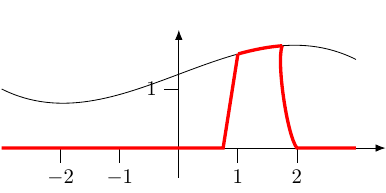Figure 4: Restricting a Function

Then we define:

 $\begin{array}{rl}{f}_{r}& ={\sum }_{k\in ℤ}{f}_{2k}\\ {f}_{i}& ={\sum }_{k\in ℤ}{f}_{2k+1}\end{array}$

And observe that $f={f}_{r}+{f}_{i}$.

The operator $J$ then takes ${f}_{2k}$ to ${f}_{2k+1}$ and ${f}_{2k+1}$ to $-{f}_{2k}$.

Let's end with the simplest example to see what's going on. Take the constant function $f=1$, as in Figure 5.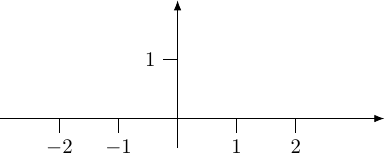Figure 5: A Constant Function

In Figure 6, the red line is the real part of $f$ and the green line is the imaginary part.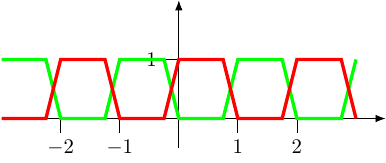Figure 6: The Real and Imaginary Parts

Applying $J$ takes the red to the green, and the green to the negative of the red, as in Figure 7.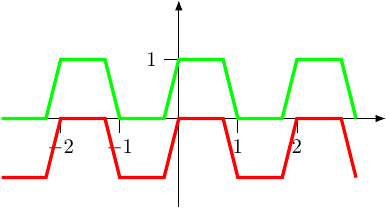Figure 7: Applying $J$ to the Real and Imaginary Parts

Recombining these results in the function shown in Figure 8.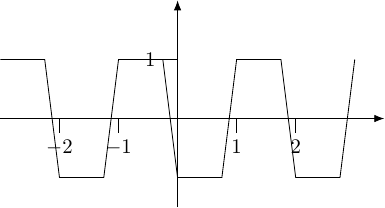Figure 8: Applying $J$ to the Constant Function

It can be illustrative to look at what happens at intermediate points, namely to look at $\mathrm{cos}\left(\theta \right)f+\mathrm{sin}\left(\theta \right)J\left(f\right)$. As the "trough" on the even intervals deepens, the "peak" on the odd intervals temporarily lifts so that when the trough is at $0$, the peak is at its height of $\sqrt{2}$.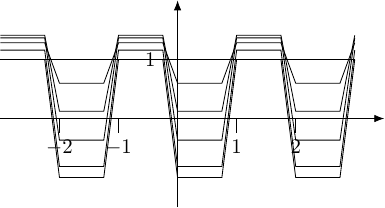Figure 9: Rotating the Constant Function

Finally, we have our answer: the operation $f↦-f$ is a rotation which can be viewed as taking place internally to the space of functions. In other words, the space of real continuous functions forms a complex vector space.